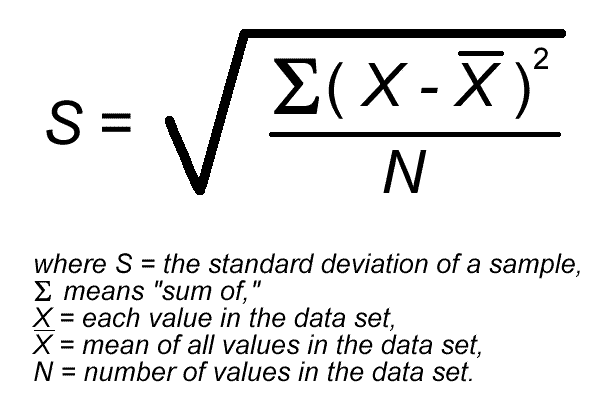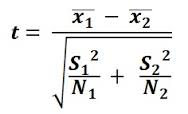Statistics in Biology

How to use excel

Excel is a spreadsheet software. This means it can be used to store and analyse data.

Data analysis included these three steps:

• 1) Draw a table (tables in excel are used for graphs and statistics, not neat tables)
• 2) Make a graph (excel will create formatted graphs for you)
• 3) Do statistics (excel will calculate all the statistics for IB biology for you)

Drawing a table:

– double click on the righthand side of a column to get the width you desire, enter the data simply by typing it in and navigating using the arrow keys

Drawing a graph:

-select your data by clicking on the top left hand corner, holding the arrow key down and dragging it over your data. If your two columns are not next to each other, it is possible to select them separately using command keys (control+command in mac)

Choose insert graph. If the graph looks wrong, click on it (control click in mac), and choose select data. You can remove all series and then add the series you want. Y values – dependent, X values – independent, and the name is what you want to call it that variable.

Error bars: To choose error bars the best way is to create a column marked ‘error bars’ in your table. You can then go into choose error bars but choose error bar options > specify values. Then you can select your error bar table to choose exactly what the error bars should be for each data point. Sometimes the error bars are different for each point e.g. if they represent standard deviations for groups of data.

How to draw a bar graph in excel (brief notes)

• Prepare your table, according to the IA student guide.
• Select the data that you wish to plot (you can select discrete columns using the control and command keys together)
• Go to insert graph, 2-d clustered column.
• If excel has plotted your x axis as a series, you will need to adjust this by clicking on your graph using control click (on the graph), and then select data.
• You can remove the unwanted series (it will usually be series one), and then select it as an x axis label.
• You should use the chart layout tab to edit the title, axese labels, gridlines (choose major and minor), and error bars.
• For error bars we may use uncertainty (for individual data readings), or standard deviation (if we are plotting means which represent sets of data)
• Prepare an error bar column, insert error bars, custom, specify value, and then highlight your error bar column in your table twice (for positive and negative)
• If x axis bars are produced, delete them.
• If your error bars are too small, they may not show up (ie if you have a small uncertainty relative to your data).

How to draw a scatter graph in excel (brief notes)

• Prepare your table, according to the IA student guide
• Select the data that you wish to plot (you can select discrete columns using the control and command keys together)
• Go to insert scatter graph, with markers
• If excel has plotted your x axis as a series, you will need to adjust this by clicking on your graph using control click (on the graph), and then select data.
• You can remove the unwanted series (it will usually be series one), and then select it as an x axis label.
• You should use the chart layout tab to edit the title, axese labels, gridlines (choose major and minor), and error bars.
• You should also add trendline, choose linear, and in option find R2 value (display it). The closer that is to 1, the better is your line of fit and the more meaningful is the relationship shown.
• For error bars we may use uncertainty (for individual data readings), or standard deviation (if we are plotting means which represent sets of data)
• Prepare an error bar column, insert error bars, custom, specify value, and then highlight your error bar column in your table twice (for positive and negative)
• If x axis bars are produced, delete them.
• If your error bars are too small, they may not show up (ie if you have a small uncertainty relative to your data).

[slideshare id=592619&doc=01-statistical-analysis-1221114089218264-9]

Task: Prepare a graph and table using the data on hummingbird length

Task: Find out if the differences between the hummingbird bill lengths are significant

Task: Choose two investigate one measurement in the classroom, and dividing the class into two groups, use the t-test to see if the differences are significant (eg. is there a significant difference in the heights between boys and girls in this class).

How to calculate Standard deviation (the long way):

1) Paste the data set into a column

2) Add a column next to it, where each cell represents the mean of that data set =average(select values)

3) Add a third column, enter a formula in the next column, for the differences between each value,= value-average

4) Add a fourth new column, to square all these differences =value^2 (this is on the keyboard)

5) Add a cell at the bottom with the total of the squared differences = sum( select the cells in column 4

6) To count the data (get N), in a cell to one side enter = count (select all the data)

6) You can now apply the formula to get the standard deviation.Calculate Standard deviation the quick way:

note of course you can skip all this by selecting =STDEV(select data). Tell me which method you preferHOW TO DO THE T-TEST

the t-test is designed to determine if the difference between the means of two groups of data is significant (is group A significantly different to group B)

Option 1: Do the t test, without using the excel formula

note: to add a table title, merge three cells above your table, and enter a title. You can select underlined.

1) Enter two groups of data in separate columns, eg. group A and group B

2) You should add a separate table named summary statistics (summary statistics describe the overall patterns in the data for example the mean), with the following columns; Group, Mean, Number, Standard deviation.

3. Calculate the mean, by =average(select A or B). Calculate the standard deviation by =STDEV(select A or B), Calculate the number= count(select A or B)

4. Find out the degrees of freedom (total number of data points in both groups -2, so = Number of A +Number of B – 2).

10) Calculate the value of t from the equation (do this bit on your calculator)X1 is the mean of A, X2 is the mean of B. S1 is the STDEV of A, S2 is the STDEV of B, N1 is the number of A, N2 is the number of B

Calculated Value of t =

11. Look in the table below, then look up the Critical value of t from the table using the degrees of freedom (step 4) and using the column 0.05 (95% confidence level, that means we are 95% confident that is a standard level of confidence for IB Bio).11) If calculated t is bigger than critical t , we reject the null hypothesis that there is no significant difference and accept the alternative hypothesis, that there is a significant difference.

If calculated t is less than critical t, we retain the null hypothesis

this is an example file which was used to follow these instructions, download it to see how the steps were carried out;

t-test workbook